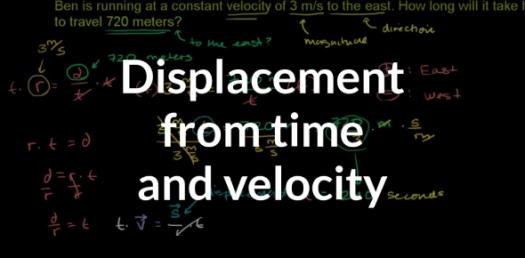# Quiz: How Much Do You Know About Displacement And Velocity?

13 Questions | Attempts: 203
ShareSettingsHow Much Do You Know About Displacement And Velocity? One of the main things you should know is that velocity is the rate at which displacement changes over time. If you want to get the velocity of an item you just need to divide the velocity by the time it moved. Take the quiz and see what else you know.

• 1.
Use the graph below for questions 1-5. What does section C represent?
• A.

• B.

Moving away from the original position

• C.

No motion

• D.

Moving towards the original position

• 2.
Which section represents the greatest average speed?
• A.

B

• B.

C

• C.

D

• D.

F

• 3.
What is the average speed from t= 6 s to t = 20 s?
• A.

- .92 m/s

• B.

.92 m/s

• C.

.79 m/s

• D.

Cannot be determined

• 4.
What is the average speed of the entire trip?
• A.

0 m/s

• B.

.55 m/s

• C.

.73 m/s

• D.

Cannot be determined from the graph

• 5.
What is the average speed of section B?
• A.

1 m/s

• B.

.25 m/s

• C.

4 m/s

• D.

-1 m/s

• 6.
Use the graph below to answer questions 6-10. What is the total distance traveled the first 40 s?
• A.

120 m

• B.

160 m

• C.

40 m

• D.

- 40 m

• 7.
What is the average speed of the first leg of the trip?
• A.

– 6 m/s

• B.

1 m/s

• C.

– 1 m/s

• D.

6 m/s

• 8.
What time interval has the lowest average velocity?
• A.

0 – 10 s

• B.

10 – 15 s

• C.

15 – 40 s

• D.

40 – 55 s

• 9.
Which statement best describes what happens at t = 15 seconds?
• A.

The object begins to speed up again at a speed greater than it was originally.

• B.

The objet begins to move farther away from its starting position.

• C.

The object changes directions.

• D.

The object does not move.

• 10.
At what time does the object change directions?
• A.

10 s

• B.

15 s

• C.

10 s & 40 s

• D.

15 s & 40 s

• 11.
A bike travels at a constant speed of 4.0 m/s for 5.0 s.  How far does it go?
• A.

20 m

• B.

.8 m

• C.

1.25 m

• D.

Not enough data to answer question

• 12.
From 3 s to 6 s the object is _________.
• A.

Slowing down

• B.

Speeding up

• C.

Has constant velocity

• D.

Changes direction

• 13.
What is the average velocity of the first 3 seconds of the trip?
• A.

.75 m/s

• B.

-3 m/s

• C.

3 m/s

• D.

1.3 m/s

## Related TopicsBack to top
×

Wait!
Here's an interesting quiz for you.